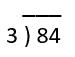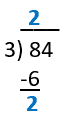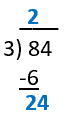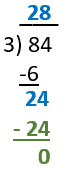# 2-digit by 1-digit Division Worksheets

## All About These 15 Worksheets

These 2-digit by 1-digit Division worksheets are designed to help students master the concept of dividing two-digit numbers by one-digit numbers. The worksheets include a variety of creative and engaging exercises to help students build their skills and confidence in division.

Each worksheet in the series includes a mix of problems with varying degrees of difficulty, designed to challenge and engage students at different skill levels. The problems require students to divide two-digit numbers by one-digit numbers with and without remainders.

These worksheets also provide ample space for students to show their work and write down their answers. This helps them develop their problem-solving skills and allows for feedback to be given by their teachers or peers.

This series is most suitable for students in grades 3 to 5. It can be used in the classroom as a supplement to the math curriculum or at home as a way for parents to help their children practice and reinforce their math skills.

Overall, these 2-digit by 1-digit Division worksheets provide a comprehensive and engaging way for students to develop their skills and confidence in dividing two-digit numbers by one-digit numbers. With these worksheets, students can build a solid foundation in math and develop the skills they need to succeed in higher-level math courses.

## How to Divide a 2-digit Number by 1-digit

Dividing a 2-digit number by a 1-digit number involves a series of steps. Here’s a step-by-step guide that teachers can share with their students to help them divide a 2-digit number by a 1-digit number using long division:

• Set up the Long Division Problem – Write the 2-digit number (dividend) inside the division symbol (also called the division bracket) and the 1-digit number (divisor) on the outside, to the left of the division symbol.
• Divide the First Digit of the Dividend by the Divisor – Check if the divisor can be divided into the first digit of the dividend. If it can, write the quotient (the result of the division) above the first digit of the dividend.
• Multiply the Divisor by the Quotient and Subtract the Result from the First Digit of the Dividend – Write the result below the first digit of the dividend, and then subtract to find the remainder.
• Bring Down the Next Digit – If there is a remainder, bring down the next digit of the dividend (the second digit in a 2-digit number) and place it beside the remainder.
• Divide the New Number by the Divisor – Divide the new number (formed by the remainder and the brought-down digit) by the divisor. Write the quotient above the dividend, next to the previous quotient digit.
• Multiply the Divisor by the Quotient and Subtract the Result from the New Number – Write the result below the new number and then subtract to find the remainder.
• Check the Remainder – If there is no remainder, the division is complete. If there is a remainder, you can either stop there or continue the division by adding a decimal point and more digits to the dividend.

Let’s divide 84 by 3 using the steps above.

Step 1: Set up the long division problemStep 2: Divide the first digit of the dividend by the divisor (8 ÷ 3 = 2)Step 3: Drop down the ones place value (4).Step 4: Divide 3 into the remaining value.  (24 ÷ 3 = 8)Step 5: Check the remainder – There is no remainder, so the division is complete.

The quotient is 28, meaning 84 divided by 3 equals 28.